1. /
2. CBSE
3. /
4. Class 12
5. /
6. Physics
7. /
8. NCERT Solutions class 12...

# NCERT Solutions class 12 physics Alternating Current### myCBSEguide App

Download the app to get CBSE Sample Papers 2023-24, NCERT Solutions (Revised), Most Important Questions, Previous Year Question Bank, Mock Tests, and Detailed Notes.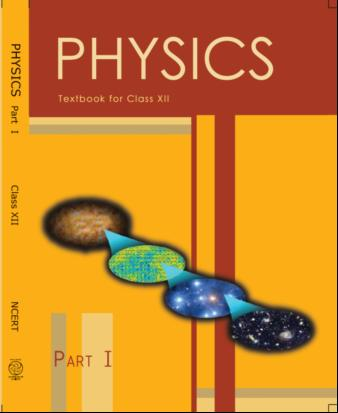## NCERT Class 12 Physics Chapter-wise Solutions

• 1 – Electric Charges and Fields
• 2 – Electrostatic Potential and Capacitance
• 3 – Current Electricity
• 4 – Moving Charges and Magnetism
• 5 – Magnetism and Matter
• 6 – Electromagnetic Induction
• 7 – Alternating Current
• 8 – Electromagnetic Waves
• 9 – Ray Optics and Optical Instruments
• 10 – Wave Optics
• 11 – Dual Nature of Radiation and Matter
• 12 – Atoms
• 13 – Nuclei
• 14 – Semiconductor Electronic: Material, Devices and Simple Circuits
• 15 – Communication Systems

## NCERT Solutions for Class 12 Chapter 7 Alternating Current

• 7.1 Introduction
• 7.2 AC Voltage Applied to a Resistor
• 7.3 Representation of AC Current and Voltage by Rotating Vectors — Phasors
• 7.4 AC Voltage Applied to an Inductor
•  7.5 AC Voltage Applied to a Capacitor
• 7.6 AC Voltage Applied to a Series LCR Circuit
• 7.7 Power in AC Circuit: The Power Factor
• 7.8 LC Oscillations
• 7.9 Transformers

## NCERT Solutions class 12 physics Alternating Current

1:A 100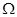resistor is connected to a 220 V, 50 Hz ac supply.

(a) What is the rms value of current in the circuit?

(b) What is the net power consumed over a full cycle?

2: (a) The peak voltage of an ac supply is 300 V. What is the rms voltage?

(b) The rms value of current in an ac circuit is 10 A. What is the peak current?

3: A 44 mH inductor is connected to 220 V, 50 Hz ac supply. Determine the rms value of the current in the circuit.

4: A 60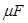capacitor is connected to a 110 V, 60 Hz ac supply. Determine the rms value of the current in the circuit.

5:  In Exercises 7.3 and 7.4, what is the net power absorbed by each circuit over a complete cycle. Explain your answer.

6:  Obtain the resonant frequency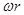of a series LCR circuit with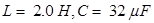and R = 10. What is the Q-value of this circuit?

7: A charged 30capacitor is connected to a 27 mH inductor. What is the angular frequency of free oscillations of the circuit?

8: Suppose the initial charge on the capacitor in Exercise 7.7 is 6 mC. What is the total energy stored in the circuit initially? What is the total energy at later time?

9: A series LCR circuit with R = 20, L = 1.5 H and C = 35is connected to a variable-frequency 200 V ac supply. When the frequency of the supply equals the natural frequency of the circuit, what is the average power transferred to the circuit in one complete cycle?

10:  A radio can tune over the frequency range of a portion of MW broadcast band: (800 kHz to 1200 kHz). If its LC circuit has an effective inductance of 200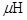, what must be the range of its variable capacitor?

[Hint: For tuning, the natural frequency i.e., the frequency of free oscillations of the LC circuit should be equal to the frequency of the radio wave.]

11:  Figure 7.21 shows a series LCR circuit connected to a variable frequency 230 V source. L = 5.0 H, C = 80, R = 40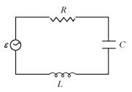(a) Determine the source frequency which drives the circuit in resonance.

(b) Obtain the impedance of the circuit and the amplitude of current at the resonating frequency.

(c) Determine the rms potential drops across the three elements of the circuit. Show that the potential drop across the LC combination is zero at the resonating frequency. 12: An LC circuit contains a 20 mH inductor and a 50capacitor with an initial charge of 10 mC. The resistance of the circuit is negligible. Let the instant the circuit is closed be t = 0.

(a) What is the total energy stored initially? Is it conserved during LC oscillations?

(b) What is the natural frequency of the circuit?

(c) At what time is the energy stored

(i) completely electrical (i.e., stored in the capacitor)? (ii) completely magnetic (i.e., stored in the inductor)?

(d) At what times is the total energy shared equally between the inductor and the capacitor?

(e) If a resistor is inserted in the circuit, how much energy is eventually dissipated as heat?

13:  A coil of inductance 0.50 H and resistance 100is connected to a 240 V, 50 Hz ac supply.

(a) What is the maximum current in the coil?

(b) What is the time lag between the voltage maximum and the current maximum?

14: Obtain the answers (a) to (b) in Exercise 7.13 if the circuit is connected to a high frequency supply (240 V, 10 kHz). Hence, explain the statement that at very high frequency, an inductor in a circuit nearly amounts to an open circuit. How does an inductor behave in a dc circuit after the steady state?

15:  A 100capacitor in series with a 40resistance is connected to a 110 V, 60 Hz supply.

(a) What is the maximum current in the circuit?

(b) What is the time lag between the current maximum and the voltage maximum?

16: Obtain the answers to (a) and (b) in Exercise 7.15 if the circuit is connected to a 110 V, 12 kHz supply? Hence, explain the statement that a capacitor is a conductor at very high frequencies. Compare this behavior with that of a capacitor in a dc circuit after the steady state.

17:  Keeping the source frequency equal to the resonating frequency of the series LCR circuit, if the three elements, L, C and R are arranged in parallel, show that the total current in the parallel LCR circuit is minimum at this frequency. Obtain the current rms value in each branch of the circuit for the elements and source specified in Exercise 7.11 for this frequency.

18: A circuit containing a 80 mH inductor and a 60capacitor in series is connected to a 230 V, 50 Hz supply. The resistance of the circuit is negligible.

(a) Obtain the current amplitude and rms values.

(b) Obtain the rms values of potential drops across each element.

(c) average power transferred to the inductor?

(d) What is the average power transferred to the capacitor?

(e) What is the total average power absorbed by the circuit? [‘Average’ implies ‘averaged over one cycle’.]

19: Suppose the circuit in Exercise 7.18 has a resistance of 15. Obtain the average power transferred to each element of the circuit, and the total power absorbed.

20 : A series LCR circuit with L = 0.12 H, C = 480 nF, R = 23is connected to a 230 V variable frequency supply.

(a) What is the source frequency for which current amplitude is maximum. Obtain this maximum value.

(b) What is the source frequency for which average power absorbed by the circuit is maximum. Obtain the value of this maximum power.

(c) For which frequencies of the source is the power transferred to the circuit half the power at resonant frequency? What is the current amplitude at these frequencies?

(d) What is the Q-factor of the given circuit?

21:  Obtain the resonant frequency and Q-factor of a series LCR circuit with L = 3.0 H, C = 27, and R = 7.4. It is desired to improve the sharpness of the resonance of the circuit by reducing its ‘full width at half maximum’ by a factor of 2. Suggest a suitable way.

(a) In any ac circuit, is the applied instantaneous voltage equal to the algebraic sum of the instantaneous voltages across the series elements of the circuit? Is the same true for rms voltage?

(b) A capacitor is used in the primary circuit of an induction coil.

(c) An applied voltage signal consists of a superposition of a dc voltage and an ac voltage of high frequency. The circuit consists of an inductor and a capacitor in series. Show that the dc signal will appear across C and the ac signal across L.

(d) A choke coil in series with a lamp is connected to a dc line. The lamp is seen to shine brightly. Insertion of an iron core in the choke causes no change in the lamp’s brightness. Predict the corresponding observations if the connection is to an ac line.

(e) Why is choke coil needed in the use of fluorescent tubes with ac mains? Why can we not use an ordinary resistor instead of the choke coil?

24:  At a hydroelectric power plant, the water pressure head is at a height of 300 m and the water flow available is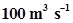. If the turbine generator efficiency is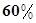estimate the electric power available from the plant (g=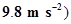.

25:  A small town with a demand of 800 kW of electric power at 220 V is situated 15 km away from an electric plant generating power at 440 V. The resistance of the two wire line carrying power is 0.5per km. The town gets power from the line through a 4000–220 V step-down transformer at a sub-station in the town.

(a) Estimate the line power loss in the form sof heat.

(b) How much power must the plant supply, assuming there is negligible power loss due to leakage?

(c) Characterise the step up transformer at the plant.

26:  Do the same exercise as above with the replacement of the earlier transformer by a 40,000-220 V step-down transformer (Neglect, as before, leakage losses though this may not be a good assumption any longer because of the very high voltage transmission involved). Hence, explain why high voltage transmission is preferred?

## NCERT Solutions for Class 12 Physics

NCERT Solutions Class 12 Physics PDF (Download) Free from myCBSEguide app and myCBSEguide website. Ncert solution class 12 physics includes text book solutions from both part 1 and part 2. NCERT Solutions for CBSE Class 12 Physics have total 20 chapters. 12 Physics NCERT Solutions in PDF for free Download on our website. Ncert physics class 12 solutions PDF and physics ncert class 12 PDF solutions with latest modifications and as per the latest CBSE syllabus are only available in myCBSEguide

To download NCERT Solutions for class 12 Physics, Chemistry, Biology, History, Political Science, Economics, Geography, Computer Science, Home Science, Accountancy, Business Studies and Home Science; do check myCBSEguide app or website. myCBSEguide provides sample papers with solution, test papers for chapter-wise practice, NCERT solutions, NCERT Exemplar solutions, quick revision notes for ready reference, CBSE guess papers and CBSE important question papers. Sample Paper all are made available through the best app for CBSE students and myCBSEguide website.Test Generator

Create question paper PDF and online tests with your own name & logo in minutes.myCBSEguide

Question Bank, Mock Tests, Exam Papers, NCERT Solutions, Sample Papers, Notes

### 1 thought on “NCERT Solutions class 12 physics Alternating Current”

1. wonderful work